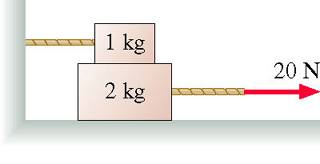# Forces and Tension

Dormin

## Homework Statement

The 1.0 kg block in the figure is tied to the wall with a rope. It sits on top of the 2.0 kg block. The lower block is pulled to the right with a tension force of 20 N. The coefficient of kinetic friction at both the lower and upper surfaces of the 2.0 kg block is mu_k = 0.380.What is the acceleration of the 2.0 kg block?

## Homework Equations

a = F/m
F_friction = mg * mu_k

## The Attempt at a Solution

I found in a previous question that the tension of the rope holding the 1kg block, and thus the force of friction on the top of the 2kg block, was 3.72N (mg * mu_k = 9.8 * .38 = 3.72).

So the frictional force on the bottom of the 2kg block:

mg * mu_k = 2 * 9.8 * .38 = 7.448

Those two forces hold back the 20N force pulling to the right, so the total force acting on it to the right is:

20 - 3.72 - 7.448 = 8.832

So using a = F/m:

a = 8.832 / 2 = 4.416 m/s^2

However, 4.416 is incorrect. Any help you could give would be appreciated. Thanks!

Last edited: